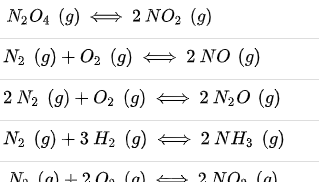# Based on Le Châtelier’s principle, increasing pressure at constant temperature in which of the following reaction will not change the concentrations of the reactants and products? a. N2O4 (g) < - > 2NO2 (g) b. N2 (g) + O2 (g) < - > 2NO (g) c. 2N2 (g) + O2 (g) < - > 2N2O (g) d. N2 (g) + 3H2 (g) < - > 2 NH3 (g) e. N2 (g) + 2O2 (g) < - > 2NO2 (g)